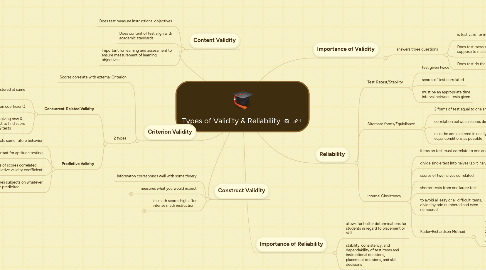# Types of Validity & Reliability

Plan your lessons and the goals of your lessons as well as including important content

Get Started. It's FreeTypes of Validity & Reliability## 2. Construct Validity

### 2.2. measures what you would expect

2.2.2. Prerequisites

### 2.3. i.e. math scores high after intense math instruction

2.3.2. Prerequisites

## 3. Criterion Validity

### 3.2. 2 types

3.2.1. Concurrent-Related Validity

3.2.1.1. measures administered at same time as measure

3.2.1.2. yields numeric value (correlation coefficient)

3.2.1.3. Deternined by giving new & established test to find score correlation of 2 tests

3.2.2. Predictive Validity

3.2.2.1. how well test predicts some future behavior

3.2.2.2. important for aptitude testing

3.2.2.3. 2 sets of scores correlated (predictive validity coefficient

3.2.2.4. measures subjects on whatever is to be predicited

## 4. Importance of Validity

### 4.1. answers three questions

4.1.1. is test valid for intended purpose?

4.1.2. Does test measure what it is suppose to measure?

4.1.3. Does test do the job it was designed to do?

## 5. Reliability

### 5.1. Test-Retest/Stability

5.1.1. test given twice

5.1.2. scores of test correlated

5.1.3. must be an appropriate time interval between tests given

### 5.2. Alternate forms/Equivilance

5.2.1. 2 forms of test equal to one another

5.2.2. correlation between scores determined

5.2.3. must be administered in nearly equal conditions as possible

### 5.3. Internal Cinsistency

5.3.1. items on test must correlate to one another

5.3.2. divide single test into halves (split-halves)

5.3.3. scores of two halves correlated

5.3.4. shorter tests from one larger test

5.3.5. to avoid all easy or all difficult items, divide by odd numbered and even numbered

5.3.6. Kuder-Richardson Method

5.3.6.1. measure to extent which items of one form have as much in common with one another as items on another test of equal value